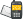It is currently 26 May 2019, 19:46### GMAT Club Daily Prep

#### Thank you for using the timer - this advanced tool can estimate your performance and suggest more practice questions. We have subscribed you to Daily Prep Questions via email.

Customized
for You

we will pick new questions that match your level based on your Timer History

Track
Your Progress

every week, we’ll send you an estimated GMAT score based on your performance

Practice
Pays

we will pick new questions that match your level based on your Timer History

#### Not interested in getting valuable practice questions and articles delivered to your email? No problem, unsubscribe here.# How many ordered pairs of positive integers (x,y) satisfy thQuestion banks Downloads My Bookmarks Reviews Important topics
Author Message
TAGS:
FounderJoined: 18 Apr 2015
Posts: 6647
Followers: 107

Kudos [?]: 1275 , given: 6017

How many ordered pairs of positive integers (x,y) satisfy th [#permalink]
Expert's post00:00

Question Stats:61% (00:44) correct38% (01:18) wrongbased on 18 sessions

How many ordered pairs of positive integers (x,y) satisfy the inequality $$2x+3y < 10$$?

A. One

B. Two

C. Three

D. Four

E. Five
[Reveal] Spoiler: OADirectorJoined: 20 Apr 2016
Posts: 868
WE: Engineering (Energy and Utilities)
Followers: 11

Kudos [?]: 645  , given: 142

Re: How many ordered pairs of positive integers (x,y) satisfy th [#permalink]
1
This post received
KUDOS
Explanation:

Here (x, y) can take integer values from 1 as they are positive integers.

So lets put the values one at a time, such that the resulting value is less than 10

1. If x = 1 and y =1, then 2*1 + 3*1 = 5 < 10

2. If x = 2 and y = 1, then 2*2 + 3*1 = 7 < 10

3. If x = 3 and y =1, then 2*3 + 3*1 = 9 < 10

4. If x = 4 and y =1, then 2*4 + 3*1 = 11 > 10 This is not possible

5. If x = 1 and y = 2, then 2*1 + 3*2 = 7 < 10

6. If x = 1 and y = 3, then 2*1 + 3*3 = 11 > 10. This is not possible

So in all, we have 4 pairs: (1, 1), (1, 2), (2, 1), (3, 1) i.e. Option D
_________________

If you found this post useful, please let me know by pressing the Kudos Button

Rules for Posting https://greprepclub.com/forum/rules-for ... -1083.html

InternJoined: 27 Mar 2019
Posts: 1
Followers: 0

Kudos [?]: 0 , given: 0

Re: How many ordered pairs of positive integers (x,y) satisfy th [#permalink]
4

Posted from my mobile deviceRe: How many ordered pairs of positive integers (x,y) satisfy th   [#permalink] 29 Mar 2019, 23:48
Display posts from previous: Sort by

# How many ordered pairs of positive integers (x,y) satisfy thQuestion banks Downloads My Bookmarks Reviews Important topicsPowered by phpBB © phpBB Group Kindly note that the GRE® test is a registered trademark of the Educational Testing Service®, and this site has neither been reviewed nor endorsed by ETS®.